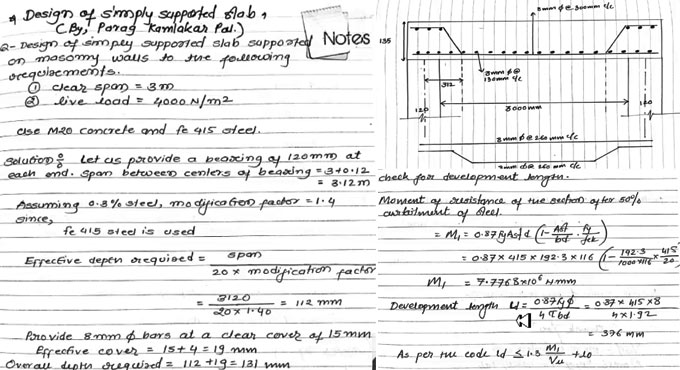# How to design any one way simply supported slabIn this construction video tutorial, you will learn how to create the design of one way slab (simply supported slab) that is standing on the masonry wall.

You will also gather information on all checks for making design of the slab.

The requirements and materials of the slab are given below :-

Clear span = 3m
Live load = 4000 N/m2
M20 concrete and fe 415 steel are used here.

The calculation is made on the following dimensions :-

A bearing of 120 mm is provided at each end. The distance among centers of bearing = 3+0.12 = 3.12 m

Necessary effective depth = Span/20 x modification factor
= 3120/20 x 1.40 = 112 mm

The bars with 8 mm diameter are arranged with clear cover of 15 mm.
Effective cover = 15 + 4 = 19 mm

Overall necessary depth = 112 + 19 = 131 mm

It is necessary to arrange an overall depth of 135 mm

So, effective depth = 135-19 = 116 mm

The Dead load of the slab will be calculated as follow :-
25 x 135 = 3375 N/m2 (floor finished with C20 mm).

Live load = 4000 N/m2
Therefore, total load = 7855 N/m2
Factor load = Wµ = 1.50 x 7855 = 11782.5 N/m2

To get more details, go through the following video tutorial.

Video Source: Parag Pal